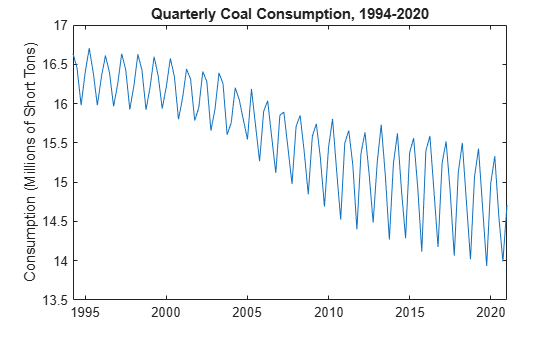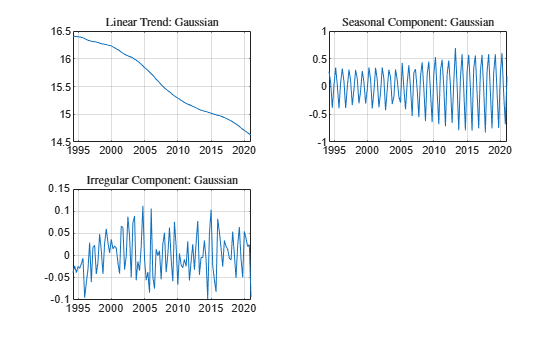# Perform Outlier Detection Using Bayesian Non-Gaussian State-Space Models

This example shows how to use non-Gaussian error distributions in a Bayesian state-space model to detect outliers in a time series. The example follows the state-space framework for outlier detection in , Chapter 14.

Robust regressions that employ distributions with excess kurtosis accommodate more extreme data compared to the Gaussian regressions. After a non-Gaussian regression (representing, for example, a linear time series decomposition model), an analysis of residuals (representing the irregular component of the decomposition) facilitates outlier detection.

Consider using a state-space model as a linear filter for a simulated, quarterly series of coal consumption, in millions of short tons, from 1994 through 2020.

Load the simulated coal consumption series `Data_SimCoalConsumption.mat`. The data set contains the timetable of data `DataTimeTable`.

`load Data_SimCoalConsumption`

Plot the coal consumption series.

```figure plot(DataTimeTable.Time,DataTimeTable.CoalConsumption) ylabel("Consumption (Millions of Short Tons)") title("Quarterly Coal Consumption, 1994-2020")```The series has a clear downward trend and pronounced seasonality, which suggests a linear decomposition model. The series does not explicitly exhibit unusually large or small observations.

### Specify State-Space Model Structure

Because the series appears decomposable, consider the linear decomposition for the observed series ${\mathit{y}}_{\mathit{t}}={\tau }_{\mathit{t}}+{\gamma }_{\mathit{t}}+{\sigma }_{3}{\epsilon }_{\mathit{t}}$, where, at time $\mathit{t}$:

• ${\mathit{y}}_{\mathit{t}}$ is the observed coal consumption.

• ${\tau }_{\mathit{t}}$ is the unobserved local linear trend.

• ${\gamma }_{\mathit{t}}$ is the unobserved seasonal component.

• ${\epsilon }_{\mathit{t}}$ is the observation innovation with mean 0. Its standard deviation depends on its distribution.

• ${\sigma }_{3}$ is the observation innovation coefficient, an unknown parameter, which scales the observation innovation.

The unobserved components are the model states, explicitly:

`$\Delta \text{\hspace{0.17em}}{\tau }_{\mathit{t}}=\Delta \text{\hspace{0.17em}}{\tau }_{\mathit{t}-1}+{\sigma }_{1}{\mathit{u}}_{1,\mathit{t}}$`

`${\gamma }_{\mathit{t}}=-{\gamma }_{\mathit{t}-1}-{\gamma }_{\mathit{t}-2}-{\gamma }_{\mathit{t}-3}+{\sigma }_{2}{\mathit{u}}_{2,\mathit{t}}$`

At time $\mathit{t}$:

• ${\mathit{u}}_{1,\mathit{t}}$ and ${\mathit{u}}_{2.\mathit{t}}$ are state disturbance series with mean 0. Their standard deviations depend on its distribution.

• ${\sigma }_{1}$ and ${\sigma }_{2}$ are the state-disturbance loading scalars, which are unknown parameters.

• Rearrange the linear trend equation to obtain ${\tau }_{\mathit{t}}=2{\tau }_{\mathit{t}-1}-{\tau }_{\mathit{t}-2}+{\sigma }_{1}{\mathit{u}}_{1,\mathit{t}}$.

The structure can be viewed as a state-space model

`${\mathit{x}}_{\mathit{t}}={\mathrm{Ax}}_{\mathit{t}-1}+\mathit{B}{\mathit{u}}_{\mathit{t}}$`

${\mathit{y}}_{\mathit{t}}={\mathrm{Cx}}_{\mathit{t}}+\mathit{D}{\epsilon }_{\mathit{t}}$,

where:

• ${\mathit{x}}_{\mathit{t}}=\left[\begin{array}{ccccc}{\tau }_{\mathit{t}}& {\mathit{x}}_{2,\mathit{t}}& {\gamma }_{\mathit{t}}& {\mathit{x}}_{4,\mathit{t}}& {\mathit{x}}_{5,\mathit{t}}\end{array}\right]\prime$, where ${\mathit{x}}_{\mathit{j},\mathit{t}}$ are dummy variables for higher order lagged terms of ${\tau }_{\mathit{t}}$ and ${\gamma }_{\mathit{t}}$.

• $\mathit{A}=\left[\begin{array}{ccccc}2& -1& 0& 0& 0\\ 1& 0& 0& 0& 0\\ 0& 0& -1& -1& -1\\ 0& 0& 1& 0& 0\\ 0& 0& 0& 1& 0\end{array}\right]$.

• $\mathit{B}=\left[\begin{array}{cc}{\sigma }_{1}& 0\\ 0& 0\\ 0& {\sigma }_{2}\\ 0& 0\\ 0& 0\end{array}\right]$.

• $\mathit{C}=\left[\begin{array}{ccccc}1& 0& 1& 0& 0\end{array}\right]$.

• $\mathit{D}={\sigma }_{3}$.

Write a function called `linearDecomposeMap` in the Local Functions section that maps a vector of parameters $\Theta$ to the state-space coefficient matrices.

```function [A,B,C,D,Mean0,Cov0,StateType] = linearDecomposeMap(theta) A = [2 -1 0 0 0; 1 0 0 0 0; 0 0 -1 -1 -1; 0 0 1 0 0; 0 0 0 1 0]; B = [theta(1) 0; 0 0; 0 theta(2); 0 0; 0 0]; C = [1 0 1 0 0]; D = theta(3); Mean0 = []; % MATLAB uses default initial state mean Cov0 = []; % MATLAB uses initial state covariances StateType = [2; 2; 2; 2; 2]; % All states are nonstationary end ```

### Specify Prior Distribution

Assume the prior distribution of each of the disturbance and innovation scalars is ${\chi }_{10}^{2}$. Write a function called `priorDistribution` in the Local Functions section that returns the log prior of a value of $\Theta$.

```function logprior = priorDistribution(theta) p = chi2pdf(theta,10); logprior = sum(log(p)); end ```

### Create Bayesian State-Space Model

Create a Bayesian state-space model representing the system by passing the state-space model structure and prior distribution functions as function handles to `bssm`. For comparison, create a model that sets the distribution of ${\epsilon }_{\mathit{t}}$ to a standard Gaussian distribution and a different model that sets the distribution of ${\epsilon }_{\mathit{t}}$ to a Student's $\mathit{t}$ distribution with unknown degrees of freedom.

```y = DataTimeTable.CoalConsumption; MdlGaussian = bssm(@linearDecomposeMap,@priorDistribution); MdlT = bssm(@linearDecomposeMap,@priorDistribution,ObservationDistribution="t");```

### Prepare for Posterior Sampling

Posterior sampling requires a good set of initial parameter values and tuned proposal scale matrix. Also, to access Bayesian estimates of state values and residuals, which compose the components of the decomposition, you must write a function that stores these quantities at each iteration of the MCMC sampler.

For each prior model, use the `tune` function to obtain a data-driven set of initial values and a tuned proposal scale matrix. Specify a random set of positive values in [0,1] to initialize the Kalman filter.

```rng(10) % For reproducibility numParams = 3; theta0 = rand(numParams,1); [theta0Gaussian,ProposalGaussian] = tune(MdlGaussian,y,theta0,Display=false);```
```Local minimum possible. fminunc stopped because it cannot decrease the objective function along the current search direction. ```
`[theta0T,ProposalT] = tune(MdlT,y,theta0,Display=false);`
```Local minimum possible. fminunc stopped because it cannot decrease the objective function along the current search direction. ```

Write a function in the Local Functions section called `outputfcn` that returns state estimates and observation residuals at each iteration of the MCMC sampler.

```function out = outputfcn(inputstruct) out.States = inputstruct.States; out.ObsResiduals = inputstruct.Y - inputstruct(end).States*inputstruct(end).C'; end ```

### Decompose Series Using Gaussian Model

Decompose the series by following this procedure:

1. Draw a large sample from the posterior distribution by using `simulate`.

2. Plot the linear, seasonal, and irregular components.

Use `simulate` to draw a posterior sample of 10000 from the model that assumes the observation innovation distribution is Gaussian. Specify the data-driven initial values and proposal scale matrix, specify the output function `outputfcn`, apply the univariate treatment to speed up calculations, and apply a burn-in period of 1000. Return the draws, acceptance probability, and output function output. `simulate` uses the Metropolis-Hastings sampler to draw the sample.

```NumDraws = 10000; BurnIn = 1000; [ThetaPostGaussian,acceptGaussian,outGaussian] = simulate(MdlGaussian, ... y,theta0Gaussian,ProposalGaussian,NumDraws=NumDraws,BurnIn=BurnIn, ... OutputFunction=@outputfcn,Univariate=true); acceptGaussian```
```acceptGaussian = 0.4620 ```

`ThetaPostGaussian` is a 3-by-1000 matrix of draws from the posterior distribution of $\Theta |\mathit{y}$. `simulate` accepted about half of the proposed draws. `outGaussian` is a structure array with 1000 records, the last of which contains the final estimates of the components of the decomposition.

Plot the components of the decomposition by calling the `plotComponents` function in the Local Functions section.

`plotComponents(outGaussian,dates,"Gaussian")`The plot of the irregular component (residuals) does not contain any residuals of unusual magnitude. Because the Gaussian model structure applies very small probabilities to unusual observations, it is difficult for this type of model to discover outliers.

### Decompose Series Using Model with t-Distributed Innovations

The $\mathit{t}$-distribution applies larger probabilities to extreme observations. This characteristic enables models to discover outliers more readily than a Gaussian model.

Decompose the series by following this procedure:

1. Estimate the posterior distribution $\Theta ,{\nu }_{\epsilon }|\mathit{y}$ by using `estimate`. Inspect the estimate of ${\nu }_{\epsilon }$.

2. Draw a large sample from the posterior distribution by using `simulate`.

3. Plot the linear, seasonal, and irregular components.

Estimate the posterior distribution of the model that assumes the observation innovations are $\mathit{t}$ distributed.

```seed = 10; % To reproduce samples across estimate and simulate rng(seed) estimate(MdlT,y,theta0T,NumDraws=NumDraws,BurnIn=BurnIn,Univariate=true);```
```Local minimum possible. fminunc stopped because the size of the current step is less than the value of the step size tolerance. Optimization and Tuning | Params0 Optimized ProposalStd ---------------------------------------- c(1) | 0.0037 0.0035 0.0011 c(2) | 0.0623 0.0627 0.0080 c(3) | 0.0370 0.0375 0.0094 Posterior Distributions | Mean Std Quantile05 Quantile95 -------------------------------------------------- c(1) | 0.0042 0.0013 0.0025 0.0066 c(2) | 0.0522 0.0079 0.0407 0.0665 c(3) | 0.0314 0.0097 0.0169 0.0490 x(1) | 14.6299 0.0308 14.5780 14.6794 x(2) | 14.6538 0.0247 14.6117 14.6935 x(3) | 0.1188 0.0491 0.0498 0.2078 x(4) | -0.6852 0.0387 -0.7538 -0.6284 x(5) | -0.1136 0.0321 -0.1647 -0.0608 ObsDoF | 4.7300 3.3612 1.5845 11.6515 Proposal acceptance rate = 35.30% ```

The `Posterior Distributions` table in the display shows posterior estimates of the state-space model parameters (labeled `c(``j``)`), final state values (labeled `x(``j``)`), and $\mathit{t}$-distribution degrees of freedom (labeled `ObsDoF`). The posterior mean of the degrees of freedom is about 5, which is low. This result suggests that the observation innovations have excess kurtosis.

Use `simulate` to draw a posterior sample of 10000 from the model that assumes the observation innovations are $\mathit{t}$ distributed. Specify the data-driven initial values and proposal scale matrix, specify the output function `outputfcn`, apply the univariate treatment to speed up calculations, and apply a burn-in period of 1000. Return the draws, acceptance probability, and output function output. `simulate` uses the Metropolis-within-Gibbs sampler to draw the sample.

```rng(seed) [ThetaPostT,acceptT,outT] = simulate(MdlT,y,theta0T,ProposalT, ... NumDraws=NumDraws,BurnIn=BurnIn,OutputFunction=@outputfcn,Univariate=true); acceptT```
```acceptT = 0.3154 ```

`ThetaPostT` is a 3-by-1000 matrix of draws from the posterior distribution of $\Theta |\mathit{y}$. `simulate` accepted about 30% of the proposed draws. `outT` is a structure array with 1000 records, the last of which contains the final estimates of the components of the decomposition.

Plot the components of the decomposition.

```plotComponents(outT,dates,"\$\$t\$\$") h = gca; h.FontSize = 8;```The plot of the irregular component shows two clear outliers around 2005.

### Local Functions

This example uses the following functions. `linearDecomposeMap` is the parameter-to-matrix mapping function and `priorDistribution` is the log prior distribution of the parameters $\Theta$.

```function [A,B,C,D,Mean0,Cov0,StateType] = linearDecomposeMap(theta) A = [2 -1 0 0 0; 1 0 0 0 0; 0 0 -1 -1 -1; 0 0 1 0 0; 0 0 0 1 0]; B = [theta(1) 0; 0 0; 0 theta(2); 0 0; 0 0]; C = [1 0 1 0 0]; D = theta(3); Mean0 = []; % MATLAB uses default initial state mean Cov0 = []; % MATLAB uses initial state covariances StateType = [2; 2; 2; 2; 2]; % All states are nonstationary end function logprior = priorDistribution(theta) p = chi2pdf(theta,10); logprior = sum(log(p)); end function out = outputfcn(inputstruct) out.States = inputstruct.States; out.ObsResiduals = inputstruct.Y - inputstruct(end).States*inputstruct(end).C'; end function plotComponents(output,dt,tcont) figure tiledlayout(2,2) nexttile plot(dt,output(end).States(:,1)) grid on title("Linear Trend: " + tcont,Interpreter="latex") h = gca; h.FontSize = 8; nexttile plot(dt,output(end).States(:,3)) grid on title("Seasonal Component: " + tcont,Interpreter="latex") h = gca; h.FontSize = 8; nexttile plot(dt,output(end).ObsResiduals) grid on title("Irregular Component: " + tcont,Interpreter="latex") end```

 Durbin, J, and Siem Jan Koopman. Time Series Analysis by State Space Methods. 2nd ed. Oxford: Oxford University Press, 2012.